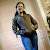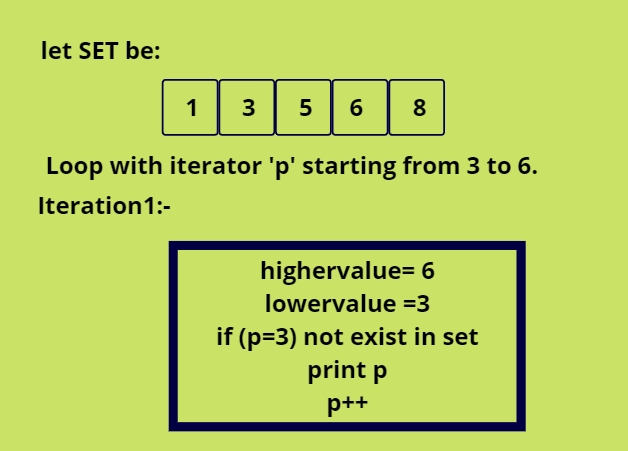New update is available. Click here to update.
Last Updated: Jun 30, 2023
Easy

# Find Missing Elements of a RangeKanak Rana
0 upvote

## Introduction

Hashing is a technique or process that uses a hash function to map keys and values into a hash table. It is done to allow for quicker access to elements. The efficiency of mapping is determined by the hash function used.Let's look into the problem to find missing elements of a range in an array.

## Problem Statement

In this article, we will be discussing the problem to find missing elements of a range.

In this, we have given an array, let's say arr[0..n-1]  of different elements and range[lower value to higher value], so we have to find all the numbers that are in the range, but not the whole array, and missing number of elements should be printed in sorted order.

### Pictorial Explanation

Let's look at the explanation of the problem statement:  To find missing elements of a range.Therefore we got ‘4’ as output as 3, 5 and 6 are already present in the set.

### Approach

Use Hashing: Make a hash table and insert all array items into it. Once all items have been placed in the hash table, traverse the range and print all missing elements of a range in an array.

#### Algorithm

1. Declare a set.

2. Traverse the array and put all the elements into the set.

3. While “i” is equal to low and “i” is less than equal to high.
• If a set does not contain “i”
• Print ‘i’.

## Code in CPP

``````/*
C++ program to find missing elements of a range.
*/

#include <bits/stdc++.h>
#include <bits/stdc++.h>
using namespace std;

// Print all elements of range [lowervalue, highervalue] that are not present in arr[0..n-1].
void Missing_number(int arr[], int a, int lowervalue, int highervalue)
{
// Insert all elements of arr[] in set
unordered_set<int> x;
for (int i = 0; i < a; i++)
x.insert(arr[i]);

// Traverse through the range a print all missing elements.
for (int p = lowervalue ; p <= highervalue; p++)
if (x.find(p) == x.end())
cout << p << " ";
}

// Driver program
int main()
{
int a;
cout<<"Entre the size of array:\n";
cin>>a;
cout<<"Enter the elements:\n";
int arr[a];
for(int i=0;i<a;i++)
{
cin>>arr[i];
}
int lowervalue, highervalue;
cout<<"Enter lower value:"\n;
cin>>lowervalue;
cout<<"Enter the higher value:\n";
cin>>highervalue;
cout<<"Missing elements are:\n";
Missing_number(arr, a, lowervalue, highervalue);
return 0;
}``````

## Code in Java

``````/*
Java program to find missing elements of a range
*/

import java.util.HashSet;
import java.util.Scanner;
public class Main
{
public static void MissingElements(int arr[], int lower, int higher)
{
HashSet<Integer> numberset = new HashSet<>();
for (int i = 0; i < arr.length; i++)
{
}
for (int i = lower; i <= higher; i++)
{
if (!numberset.contains(i))
{
System.out.print(i + " ");
}
}
}

public static void main(String[] args)
{
int n;
int [] given_arr;
Scanner input = new Scanner(System.in);
System.out.println("Enter the size of the arrray : \n");
n = input.nextInt();
given_arr = new int[n];
System.out.println("Enter the input digits for array : \n");
for(int i=0;i<n;i++)
{
int temp = input.nextInt();
given_arr[i] = temp;
}
int lower, higher;
System.out.println("Enter the lower value:\n");
lower = input.nextInt();
System.out.println("Enter the higher value:\n");
higher = input.nextInt();
MissingElements(given_arr, lower, higher);
}
}``````

## Code in Python

``````# A hashing-based Python3 program to find missing elements of a range in  an array

# Print all elements of range  [lower, higher] that are not present in arr[0..n-1]
def Missingnumber(arr, a, lower, higher):
# Insert all elements of arr[] in set
x = set(arr)
# Traverse through the range
# and print all missing elements
print("Missing numbers are:")
for p in range(lower, higher + 1):
if p not in x:
print(p, end=' ')
return

# Driver Code
arr = []
a = int(input("Enter the length of array:"))
print("Enter the Elements of array:")
for x in range(0, a):
z = int(input())
arr.append(z)
lower = int(input("Enter the lower value: "))
higher = int(input("Enter the higher value: "))
Missingnumber(arr, a, lower, higher)``````

## Complexity Analysis

### Time Complexity

O(n + (higher-lower+1)) where “n” is the number of elements present in the array, “high” and “low” is the input provided.

### Space Complexity

O(n), in the worst case, if all elements are distinct. We’ll have to store all the elements. Thus making the algorithm requires linear time.

### What are the advantages of using hash values?

When compared to other data structures, hashing offers a more flexible and secure way to retrieve data. Compared to searching for lists and arrays, it is faster.

### What is the key in hashing?

The raw data to be hashed is the hashing key. The hashing algorithm is the function that performs to convert the hash key to the hash value. The hash value is the result of passing the hash key through the hashing algorithm.

### What is the time complexity of hashing?

O(1) time

The required location is accessed in O(1) time complexity, and the hash key is calculated as usual in O(1) time complexity (1). In the ideal scenario, the element is directly inserted into the hash table because the key indicates a free space there. So, O is the overall complexity (1).

### What are the problems with hashing?

• Table dimensions.
• The hash function.
• Collision resolution

### In Python, how can I determine which elements are most frequent?

Use Python Counter, which provides a count for each element in a list. So, using the most common() method, we can easily identify the element that is used the most.

## Conclusion

In this article, we have extensively discussed the problem to find missing element of a range in an array. We hope this blog has helped you understand this problem very well.